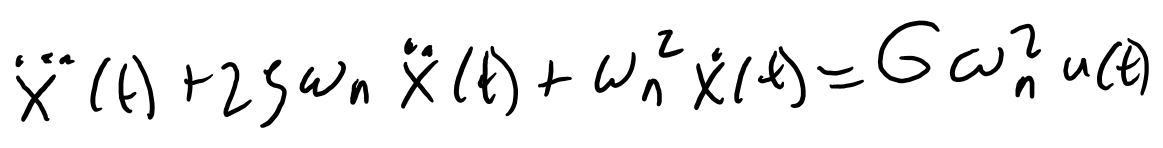Robotics 2
Time Response Modeling
QuizQuestion 3:  Suppose you have captured a step response that looks like this.  What is the value of the time constant, tau, in units of seconds?
Question 4:  Suppose you have captured a step response that looks like this.  What is the value of the damping ratio, zeta?Question 1:  Which of these equations represents the relationship between the input signal and the output signal for a first-order system?Question 2:  Which of these equations represents the relationship between the input signal and the output signal for a second-order system?Question 6: In the second video, after watching the response of the stepper and camera, I immediately assumed that the system is a second-order system.  Which of these might explain how I knew that the system was second-order?
Questions 7-10: Assuming that the gain of the stepper and camera system in the second video is 1, fill in the other numbers for the complete system model.  Use the numbers we found in the video, and round each number to 2 decimal places.
Question 5:  For the step response in Question 4, what is the value of the natural frequency, omega n, in units of radians per second?
The system has overshoot.  Only a second-order system has overshoot, so that means the system must be second-order.
The system has a gain.  Only a second-order system has a gain, so that means the system must be second-order.
The system has a time constant.  Only a second-order system has a time constant, so that means the system must be second-order.
The system has proportional control.  Only a second-order system can have proportional control, so that means the system must be second-order.